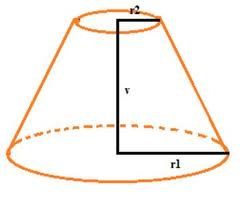# Deviation 70434

Express the surface and volume of the cut cone using its side s if the following applies to the radii of the figures r1 and r2: r1> r2, r2 = s, and if the deviation of the side from the plane of the base is 60 °.

Result

S = (Correct answer is: 18.0642 s^2)V = (Correct answer is: 4.3078 s^3)### Step-by-step explanation:Did you find an error or inaccuracy? Feel free to write us. Thank you!

Tips for related online calculators
Need help calculating sum, simplifying, or multiplying fractions? Try our fraction calculator.
Do you know the volume and unit volume, and want to convert volume units?